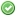rfc:list_reference_assignment

PHP RFC: list() Reference Assignment

Introduction

PHP has had list() assignment and reference assignment for a long time. However, it is not currently possible to use reference assignment with list(). This RFC proposes this new syntax to cover this. The original requests for this feature date back at least 16 years now . We will be focusing on the formers syntax proposal for this RFC.

Proposal

Under this proposal, a new syntax is introduced:

\$array = [1, 2];
list(\$a, &\$b) = \$array;

This would be equivalent to the following:

\$array = [1, 2];
\$a = \$array;
\$b =& \$array;
/* Note; []= syntax works the same, so the following is functionally equivalent to the example */
[\$a, &\$b] = \$array;

Of course, this works just like list() normally does, so you can use it with nested list() and skip values as well:

\$array = [1, 2, 3, [3, 4]];
list(&\$a, \$b,, list(&\$c, \$d)) = \$array;
var_dump(\$array);
/*
array(4) {
=>
&int(1)
=>
int(2)
=>
int(3)
=>
array(2) {
=>
&int(3)
=>
int(4)
}
}
*/

It also works with foreach():

\$array = [[1, 2], [3, 4]];
foreach (\$array as list(&\$a, \$b)) {
\$a = 7;
}
var_dump(\$array)
/*
array(2) {
=>
array(2) {
=>
int(7)
=>
int(2)
}
=>
array(2) {
=>
&int(7)
=>
int(4)
}
}
*/

(notice here that the reference exists on \$array since \$a is still in scope after the foreach())

The predominant advantage of adding support for this is that it allows you to use reference assignment for multiple variables at once, which is not currently possible. The syntax here is different from the traditional assignment syntax which places the & before the right-side value, not the left-side value; the advantage here is that you can reference assign some, but not all of the variables in list().

The RFC also takes into account the possibility of objects being the r-val of the assignment implementing ArrayAccess.

class RefArr implements ArrayAccess {
private \$s = [];
function __construct(array \$a) { \$this->s = \$a; }
function offsetSet (\$k, \$v) { \$this->s[\$k] = \$v; }
function &offsetGet (\$k) { return \$this->s[\$k]; }
function offsetExists (\$k) { return isset(\$this->s[\$k]); }
function offsetUnset (\$k) { unset(\$this->s[\$k]); }
}
\$a = new RefArr(array(1, array(2, 3)));
list(&\$one, list(\$two, &\$three)) = \$a;
\$one++;
var_dump(\$a)
/*
object(RefArr)#1 (1) {
["s":"RefArr":private]=>
array(2) {
=>
&int(2)
=>
array(2) {
=>
int(2)
=>
&int(3)
}
}
}
*/

Backward Incompatible Changes

This introduces no backwards incompatible changes.

PHP 7.2

RFC Impact

To Opcache

Maybe, I don't know enough about opcache to say if changing current ops by adding an extended value would impact.

Proposed Voting Choices

Voting starts on 2017-02-06 17:30 UTC and closes on 2017-02-22 20:00 UTC

2/3 Vote Required Vote Yes to allow reference assignments as part of list() assignments Vote No to maintain current functionality

list() Reference Assignments
Real name Yes No
ashnazg (ashnazg)bishop (bishop)bwoebi (bwoebi)daverandom (daverandom)dm (dm)fmk (fmk)galvao (galvao)hywan (hywan)joey (joey)kguest (kguest)leigh (leigh)levim (levim)lstrojny (lstrojny)mariano (mariano)ocramius (ocramius)peehaa (peehaa)pollita (pollita)salathe (salathe)sammyk (sammyk)stas (stas)svpernova09 (svpernova09)tpunt (tpunt)trowski (trowski)zeev (zeev)Final result: 17 7
This poll has been closed.

Change Log

• v2.0 - Commandeered for revival for PHP 7 implementation.
• v1.0(and prior) - Initial concept raised for PHP 5.x. Was withdrawn in 2014.

Page Tools﻿